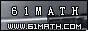Today is . WELCOME: huohai3983/44663  Home | Reg Login | All user | setHomePage | BookmarkHome | Math Test Online | Addition | Subtraction | Multiplication | Division | Time | Worksheet(JPG/PDF) | CAI | Flash Games |Recommendation articleArticle searchHome--maths--Third Grade--Add three 2-digit Numbers

How to add three numbers (for example 45 + 82 + 67).

• Place the numbers in a column so that the tens' place digits and ones' place digits are lined up. Draw a line under the bottom number.
```45
82
67
```
• Add the three ones' place digits. (5 + 2 + 7 = 14). This sum is a two-digit number, so place a one above the tens' place column and place the four below the line in the ones' place column.
```1
45
82
67
4
```
• Add the numbers in the tens' place column (1 + 4 + 8 + 6 = 19) and place the answer below the line and to the left of the ones' place sum
```  1
45
82
67
194```
• If the sum of the ones' place digits is less than ten no number is placed above the tens' place column

#### Find the Sum of the three Numbers.

 =

2006-10-17 16:02:05
Viewed 2997 times CloseGreatmathsites